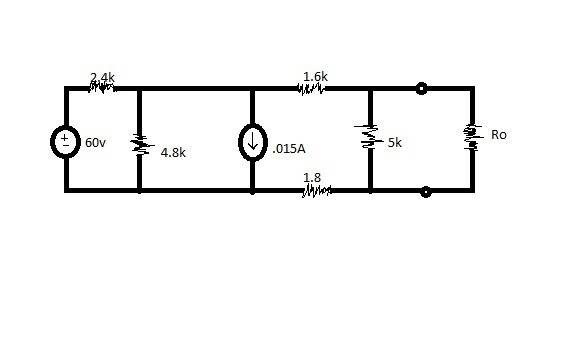# Maximum Power Transfer

## Homework Statement

Hey guys,
I have been struggling on this problem for a while now and was hoping I could get a little help

What is wanted is the maximum value of Ro for maximum power.## The Attempt at a Solution

Knowing that the Maximum power transfer to Ro would be when Rth=Ro, I am just looking for a Thevenin equivalent.

I have gone through and did the Rth to be equal to 2.5k omhs.
I did Mesh Analysis and got the following 2 equations and 1 controlling equation:
2400(i1)+60+4800(i1-i2)=0
4800(i2-i1)+1800(i3)+1600(i3)=0
(i3)+(i2)=.015Amps
[/B]
Which I reduce to:
7200(i1)-4800(i2)=-60
-4800(i1)+4800(i2)+3400(i3)=0
(i3)=.015-(i2)

Knowing that I want to get the (i3) which would tell me the current going through the Thevenin equivalent. I have also used Current Node Analysis and would want to find the Voltage drop through the 5k resistor. The answers supplied should be 4V and .0016Amps.

Any help would be great, Thanks.

## Answers and Replies

gneill
Mentor
Hi Matthew R, Welcome to Physics Forums.

In your first mesh equation you have given the 60 V source the same sign as the potential drops. This is not correct; if you are summing potential drops around a clockwise traversal of the loop; the 60 V should be negative.

In your second mesh equation (a supermesh incorporating loops two and three due to the current source between them), you haven't taken into account the 5k resistor in the third loop.

Ah I see that with the voltage source.

When creating the current with a current Thevenin equivent, would it be a short across the Ro thus bypassing the 5k resistor?

Even doing so still doesnt put the voltage at the 5k resistor at 4V

NascentOxygen
Staff Emeritus
Science Advisor
Ah I see that with the voltage source.

When creating the current with a current Thevenin equivent, would it be a short across the Ro thus bypassing the 5k resistor?
The "short-circuited output" condition will mean no current goes through the 5k.

The "open-circuited output" condition is where you determine the Thévenin voltage.

Even doing so still doesnt put the voltage at the 5k resistor at 4V

I get the open-circuit output gives me the Thevenin voltage which would be the voltage drop across the 5k resistor and that the short circuit has no current going through the 5k resistor. I am however not getting the correct voltage or current

NascentOxygen
Staff Emeritus
Science Advisor
Post your working so we can see whether there's a mistake.

Should we understand the resistor marked 1.8 to be Ω or kΩ?

rude man
Homework Helper
Gold Member
You could alternatively just compute the current io in Ro, the maximize i2oRo wrt to Ro to find Ro.

I would almost suggest doing a Norton circuit instead, with a source transform at the 60V source, it could be really easy. Just a thought though.